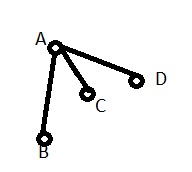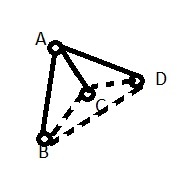# COTA - Editorial

Tester: Tasnim Imran Sunny
Editorialist: Lalit Kundu

MEDIUM-HARD

### PROBLEM:

There will be two teams (Radiant and Dire) each consisting of N players. There are 2N players. One team has players (1,3,…2N-1) and other team has (0,2,…2N-2). There teams have not yet been assigned to Radiant or Dire. We have both options. Some of the players are friends and power of their friendship is given. Some elite players are to be selected (atleast 1). Elite player cannot have a friendship with a non-elite player. Friendships can be broken.
Each Radiant member should have odd number of elite friends and each Dire member should have even number of elite friends (possibly zero).
We need to minimise the total friendship of elite players ie. sum of friendships of elite players.

### EXPLANATION:

Since each Radiant player should have elite number of friends (ie. at least 1) and non-elite players cannot be friends with elite players, all Radiant players should be elite.

We will first fix team with players (0,2,…2N-2) as Radiant and find the minimum total friendship of elite players and then do vice-versa and take the minimum of the two cases.

Now, there can be two cases.

1. There are no elite players in Dire side.
2. There are elite players in Dire side.

In case 1,
Each Dire node should have even friends (possibly zero), so we will remove edges from each vertex in Dire side. We are left with N Radiant elite nodes. We have to keep minimum weight edges and in a way such that all these nodes have odd degrees. As we are trying to minimize total edge cost, we can connect each Radiant node with another single Radiant node and remove other incident edges, if necessary. So we are trying to give matching in a general graph. N is even for this reason. Otherwise no matching would exist.

Example:
Suppose we have this graph of radiant nodes:If we do a matching in this graph, we cannot include all nodes. So we make a complete graph of the same graph. Those nodes which don’t have any edge between them are now connected by a edge of weight equivalent to shortest path between them.
So, we do a matching on this graph:So, we get a matching (a–b) and (c–d). Here (c–d) is equivalent to the path (c–a--d).

Now, case 2, we have some elite players in Dire side. So, the general idea here would be to why not just ignore the Dire nodes( like convert this case to case 1), because we are reducing edges here and we have to reduce total friendship of elite nodes. But this would be wrong, here is an example. Suppose two Radiant nodes a and b are connected (a–b) in one arrangement. We have another arrangement (a–c--d–b) where c,d are Dire elite nodes. It is possible that second arrangement is less costly than the first one.
So what we do here is build a complete graph of all the nodes (both Radiant and Dire) and do a general matching only of Radiant Nodes. Since we are matching only Radiant Nodes, there will exist a total of (N/2) paths between them. Some Dire nodes may occur in those paths but those will have degree 2 and hence satisfy the constraints.

To build the complete graph, we can use any shortest path algorithm like Floyd-Warshall etc.

Now we come to the part of minimum weighted matching in a general graph. We can do this by Dynamic Programming. We have a graph of N nodes and we need to do matching such that no two edges share same vertex and we have to minimise the sum of weight of final edges.
We keep a bitmask M whose i’th bit is 1 if the node with index i has been already matched.

Pseudo code:

``````def matching(mask):
return 0
ret=inf
for i=0 to n-1:
for j=i+1 to n-1:
if i'th and j'th bit of mask both are 0:
ret=min(ret, solve( mask | (1<<i) | (1<<j) ) + dist(i,j) ) // we mark i'th and j'th bits as 1.
break  // order of matching doesn't matter, so we can break here
return ret
``````

We can use memoization in above pseudo code. So complexity here would be O ( N * (2^N) ). Testcases were set in a way that O ( N * N * (2^N) ) gives TLE.

### AUTHOR’S AND TESTER’S SOLUTIONS:

Author’s solution can be found here.
Tester’s solution can be found here.

2 Likes

Not sure if this is said at any point, but I feel it should be said explicitly: there is no point in not making all players elite (and it makes thinking about the problem much easier).

1 Like

@dj3500: This was not mentioned explicitly. I think solver should figure out that himself.

Yes, but I mean in the editorial. For example, there really are not two cases.

1 Like
//